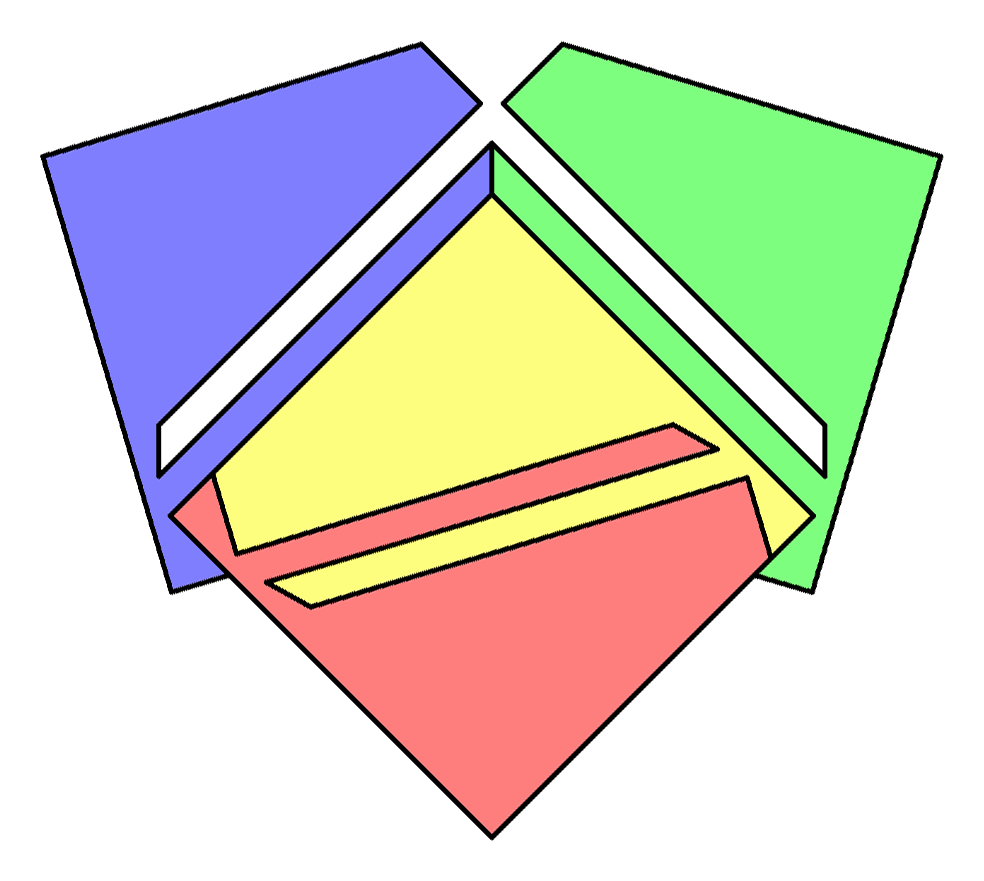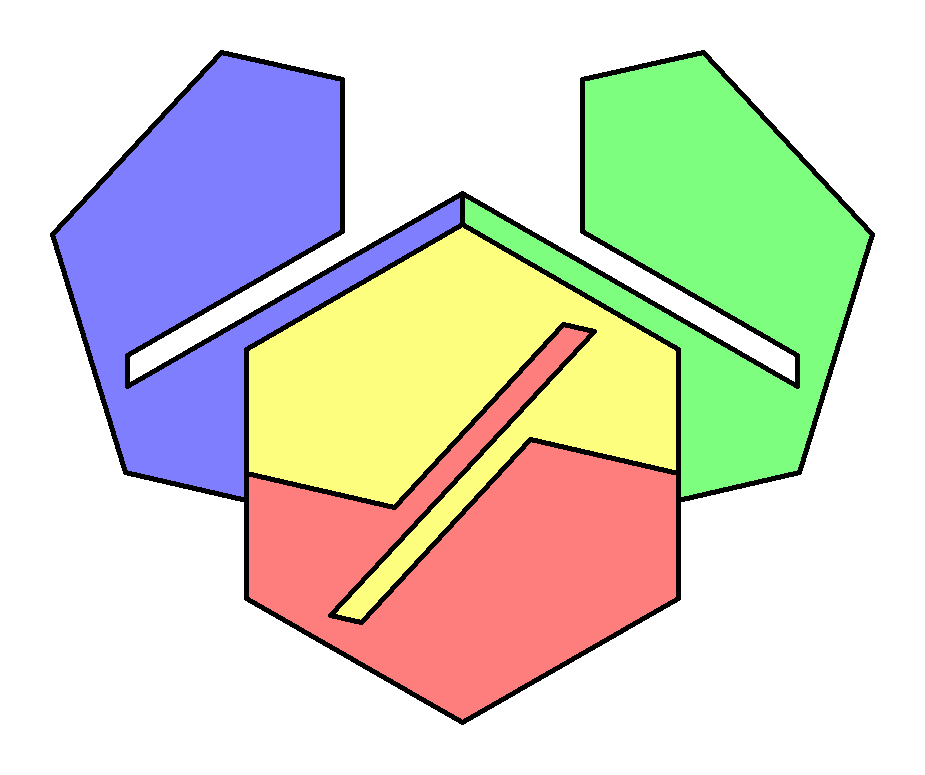11. Can two tiles of a Tetrad form a regular polygon?

Odd number of edges
As n-gons with odd n are not point symmetric, the two tiles which form the n-gon have be mirror images of one another. But as the tiles cannot be convex there has to be a hole in the n-gon. The whole tetrad has at least two holes.

Even number of edges
Two tiles form a n-gon with a point symmetric structure of the dissection lines. The shape of the whole tetrad is mirror symmetric and has no holes. For each even n there are two essentially different ways: the two tiles outside the n-gon touch in the middle of an edge or in a vertex of the n-gon (see n = 4)

n = 3   Trianglen = 4   Squaren = 5   Pentagonn = 6   Hexagonn = 7   Heptagonn = 8   OctagonPrevious Question Tetrads Contents Next Question